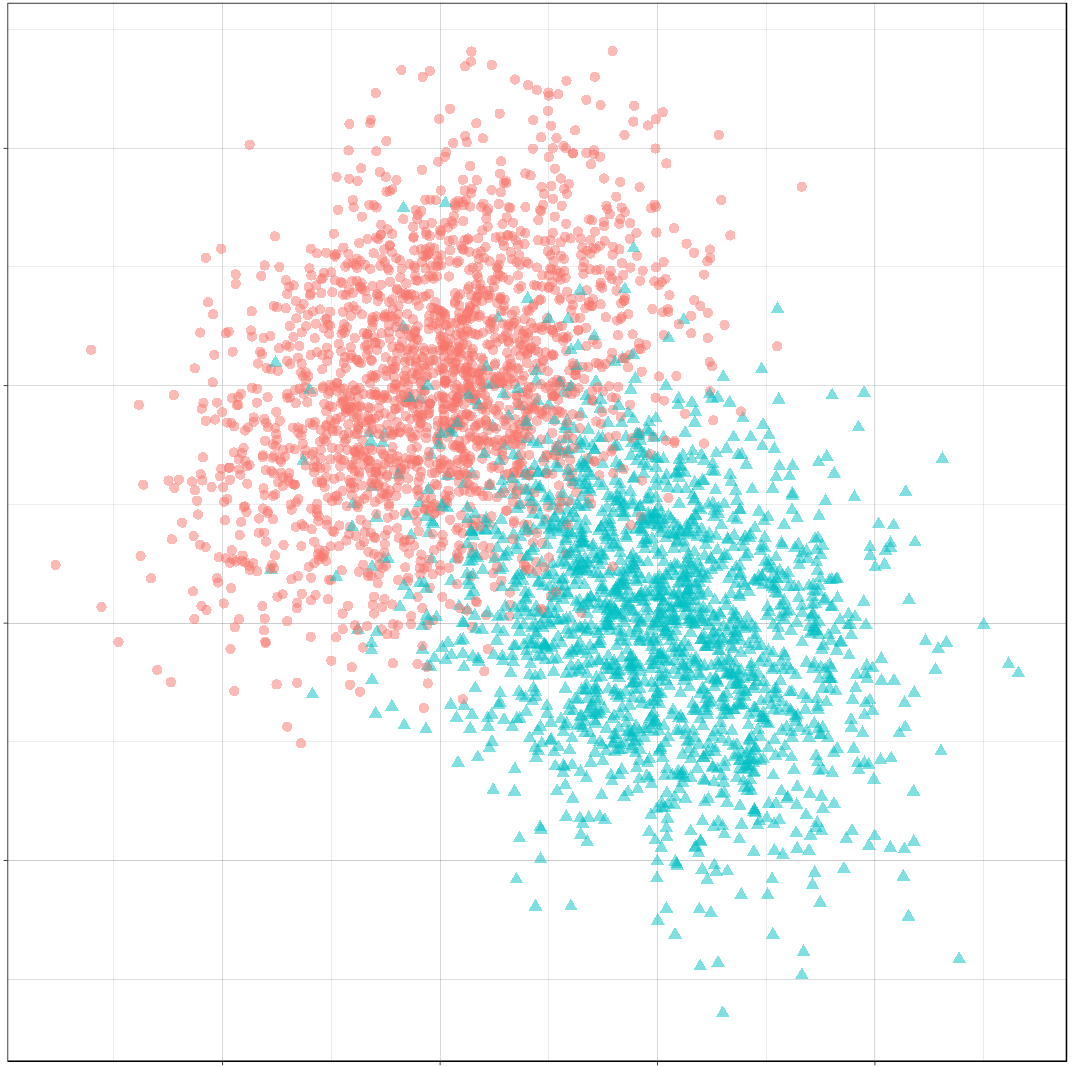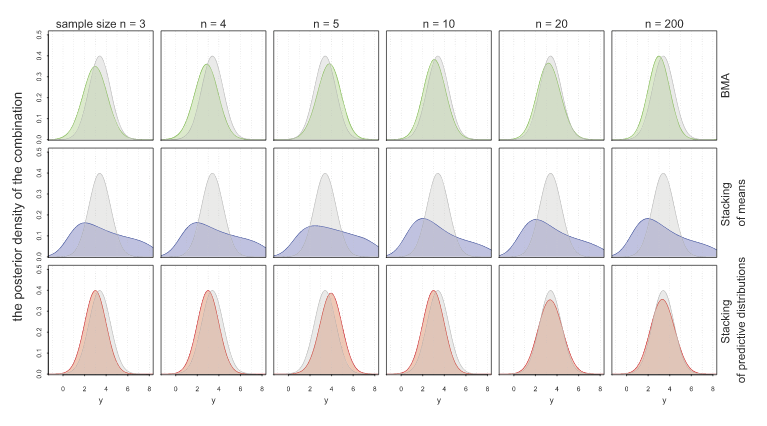# Articles by Ryan Holbrook

### Optimal Decision Boundaries

January 8, 2020 |

Introduction Over the next few posts, we will investigate decision boundaries. A decision boundary is a graphical representation of the solution to a classification problem. Decision boundaries can help us to understand what kind of solution might be appropriate for a problem. They can also help us to understand the ...### What I’m Reading 1: Bayes and Means

October 3, 2019 |

Bayesian Aggregation Yang, Y., & Dunson, D. B., Minimax Optimal Bayesian Aggregation 2014 (arXiv) Say we have a number of estimators $$\hat f_1, \ldots, \hat f_K$$ derived from a number of models $$M_1, \ldots, M_K$$ for some regression problem $$Y = f(X) + \epsilon$$, but, as is the nature of things ...